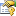# RungeKuttaAlgorithm Class

The basic Runge-Kutta integration algorithm used by the integrators, it's Butcher Tableau, and the derivative information computed during integration.

y[n+1] = y[n] + h * Sum(i=0 to s){ b[i]*k[i] }

k[i] = f(t[n] + c[i]*h, y[n] + Sum(j=0 to i-1){ a[i,j] * k[j] } )Inheritance Hierarchy
SystemObject

Assembly:  AGI.Foundation.Core (in AGI.Foundation.Core.dll) Version: 23.2.417.0 (23.2.417.0)Syntax
```public class RungeKuttaAlgorithm : IThreadAware,
ICloneWithContext```

The RungeKuttaAlgorithm type exposes the following members.Constructors
NameDescriptionRungeKuttaAlgorithm(RungeKuttaAlgorithm, CopyContext)
Initializes a new instance as a copy of an existing instance.RungeKuttaAlgorithm(Double, Double, Double)

Initializes a new instance of a Runge-Kutta algorithm with the given Butcher Tableau.

y[n+1] = y[n] + h * Sum(i=0 to s){ b[i]*k[i] }

k[i] = f(t[n] + c[i]*h, y[n] + Sum(j=0 to i-1){ a[i,j] * k[j] } )

TopProperties
NameDescriptionCoefficients
Gets the coefficients "a". These represent the factors multiplying the derivatives to provide the intermediate state information.DerivativeFunction
Gets the delegate which defines the first order differential equation representing the derivative of the dependent variables as a function of independent variable and dependent variables.Derivatives
Gets the derivative information used in the algorithm. These represent the values of "k" in the Butcher Tableau for each dependent variable, with the first index representing the stage "i" and the second representing the index of the dependent variable.IsThreadSafe
Gets a value indicating whether the methods on this instance are safe to call from multiple threads simultaneously.NumberOfStages
Gets the total number of stages in this instance of the Runge-Kutta integrator.Stages
Gets the coefficients "c". These represent the factors multiplying the step size by which to advance the independent variable at each stage.Weights
Gets the coefficients "b". These represent the coefficients of the "k" stages summed together to represent the slope.WorkingArray
Gets the working array used as a means to efficiently update the intermediate state information when calling the derivative DerivativeFunction.
TopMethods
NameDescriptionClone
Clones this object using the specified context.Equals
Determines whether the specified object is equal to the current object.
(Inherited from Object.)Finalize
Allows an object to try to free resources and perform other cleanup operations before it is reclaimed by garbage collection.
(Inherited from Object.)GetHashCode
Serves as the default hash function.
(Inherited from Object.)GetType
Gets the Type of the current instance.
(Inherited from Object.)Initialize
Initializes the derivative DerivativeFunction and the working arrays used during integration.Integrate
Compute an integration step and update the final dependent variables and store the Derivatives.MemberwiseClone
Creates a shallow copy of the current Object.
(Inherited from Object.)ToString
Returns a string that represents the current object.
(Inherited from Object.)
TopSee Also